# Search

About 110 Search Results Matching Types of Worksheet, Worksheet Section, Generator, Generator Section, Grades matching 4th Grade, Similar to Printable Kids Crossword Puzzles, Page 3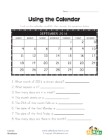## Using a Calendar Worksheet

Look at the calendar and then answer the questions...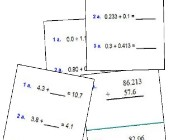## Adding Decimals Worksheets

Help kids practice decimal addition with the follo...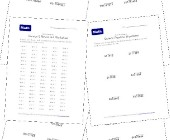## Long Division Worksheets

Help kids practice long division with this set of ...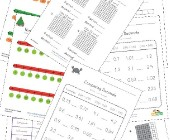## Decimal Worksheets

This collection of free decimal worksheets will he...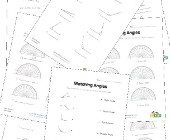## Angles Worksheets

Help kids learn about angles with this collection ...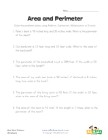## Area and Perimeter Word Problems Worksheet

Solve the 6 area and perimeter word problems in th...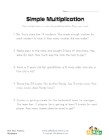## Simple Multiplication Word Problems Worksheet

Use simple multiplication to solve the 5 word prob...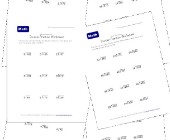## Division Practice Worksheets

This collection of division practice worksheets is...